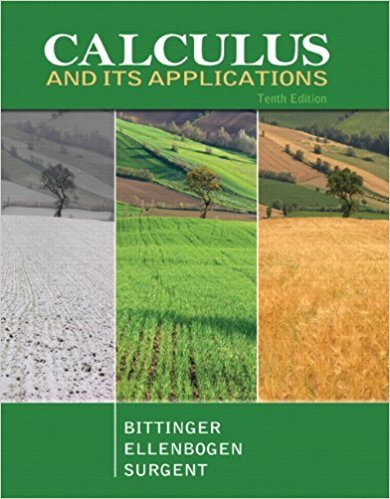×
×

# Solutions for Chapter 2: Applications of Differentiation## Full solutions for Calculus and Its Applications | 10th Edition

ISBN: 9780321694331Solutions for Chapter 2: Applications of Differentiation

Solutions for Chapter 2
4 5 0 310 Reviews
28
2
##### ISBN: 9780321694331

Chapter 2: Applications of Differentiation includes 58 full step-by-step solutions. This expansive textbook survival guide covers the following chapters and their solutions. Since 58 problems in chapter 2: Applications of Differentiation have been answered, more than 25792 students have viewed full step-by-step solutions from this chapter. This textbook survival guide was created for the textbook: Calculus and Its Applications, edition: 10. Calculus and Its Applications was written by and is associated to the ISBN: 9780321694331.

Key Calculus Terms and definitions covered in this textbook
• Absolute maximum

A value ƒ(c) is an absolute maximum value of ƒ if ƒ(c) ? ƒ(x) for all x in the domain of ƒ.

P(A or B) = P(A) + P(B) - P(A and B). If A and B are mutually exclusive events, then P(A or B) = P(A) + P(B)

• Blind experiment

An experiment in which subjects do not know if they have been given an active treatment or a placebo

• Cofunction identity

An identity that relates the sine, secant, or tangent to the cosine, cosecant, or cotangent, respectively

• Coterminal angles

Two angles having the same initial side and the same terminal side

• Difference of two vectors

<u1, u2> - <v1, v2> = <u1 - v1, u2 - v2> or <u1, u2, u3> - <v1, v2, v3> = <u1 - v1, u2 - v2, u3 - v3>

• Equal matrices

Matrices that have the same order and equal corresponding elements.

• Origin

The number zero on a number line, or the point where the x- and y-axes cross in the Cartesian coordinate system, or the point where the x-, y-, and z-axes cross in Cartesian three-dimensional space

• Piecewise-defined function

A function whose domain is divided into several parts with a different function rule applied to each part, p. 104.

• RRAM

A Riemann sum approximation of the area under a curve ƒ(x) from x = a to x = b using x1 as the right-hand end point of each subinterval.

• Semiperimeter of a triangle

One-half of the sum of the lengths of the sides of a triangle.

• Solution set of an inequality

The set of all solutions of an inequality

• Standard form of a polar equation of a conic

r = ke 1 e cos ? or r = ke 1 e sin ? ,

• Sum of complex numbers

(a + bi) + (c + di) = (a + c) + (b + d)i

A graph in which (-x, y) is on the graph whenever (x, y) is; or a graph in which (-r, -?) or (r, ?, -?) is on the graph whenever (r, ?) is

• Unit circle

A circle with radius 1 centered at the origin.

• Vector equation for a line in space

The line through P0(x 0, y0, z0) in the direction of the nonzero vector V = <a, b, c> has vector equation r = r0 + tv , where r = <x,y,z>.

• Vertex of a parabola

The point of intersection of a parabola and its line of symmetry.

• Vertical stretch or shrink

See Stretch, Shrink.

• y-coordinate

The directed distance from the x-axis xz-plane to a point in a plane (space), or the second number in an ordered pair (triple), pp. 12, 629.

×